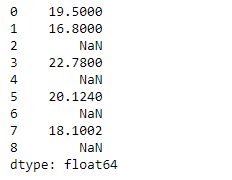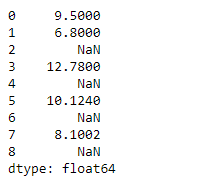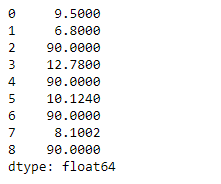Open in App
Not now

# Python | Pandas Series.subtract()

• Last Updated : 05 Feb, 2019

Pandas series is a One-dimensional ndarray with axis labels. The labels need not be unique but must be a hashable type. The object supports both integer- and label-based indexing and provides a host of methods for performing operations involving the index.

Pandas` Series.subtract()` function basically perform subtraction of series and other, element-wise (binary operator sub). It is equivalent to `series - other`, but with support to substitute a fill_value for missing data in one of the inputs.

Syntax: Series.subtract(other, level=None, fill_value=None, axis=0)

Parameter :
other : Series or scalar value
fill_value : Fill existing missing (NaN) values, and any new element needed for successful Series alignment, with this value before computation.
level : Broadcast across a level, matching Index values on the passed MultiIndex level

Returns : Series

Example #1 : Use `Series.subtract()` function to subtract a scalar from the given Series object element-wise.

 `# importing pandas as pd``import` `pandas as pd`` ` `# Creating the Series``sr ``=` `pd.Series([``19.5``, ``16.8``, ``None``, ``22.78``, ``None``, ``20.124``, ``None``, ``18.1002``, ``None``])`` ` `# Print the series``print``(sr)`

Output :Now we will use `Series.subtract()` function to perform subtraction of the series with a scalar element-wise.

 `# subtract all the elements of the ``# series by 10``sr.subtract(``10``)`

Output :As we can see in the output, `Series.subtract()` function has successfully subtracted all the elements of the given Series object by 10. Notice no subtraction has been performed on the missing values.

Example #2 : Use `Series.subtract()` function to subtract a scalar from the given Series object element-wise. Also replace the missing values by 100.

 `# importing pandas as pd``import` `pandas as pd`` ` `# Creating the Series``sr ``=` `pd.Series([``19.5``, ``16.8``, ``None``, ``22.78``, ``None``, ``20.124``, ``None``, ``18.1002``, ``None``])`` ` `# Print the series``print``(sr)`

Output :Now we will use `Series.subtract()` function to perform subtraction of the series with a scalar element-wise. We will replace the missing value in our series object by 100.

 `# subtract all the elements of the ``# series by 10 and also fill 100 at``# the place of missing values.``sr.subtract(``10``, fill_value ``=` `100``)`

Output :As we can see in the output, `Series.subtract()` function has successfully subtracted all the elements of the given Series object by 10. Notice how we have substituted 100 at the places of the missing values.

My Personal Notes arrow_drop_up